Fractions on a Number Line 1
Set 1 (20 Questions)
Identify and represent on a number line decimals, fractions, mixed numbers, and positive and negative integers.
From Mr. Anker Tests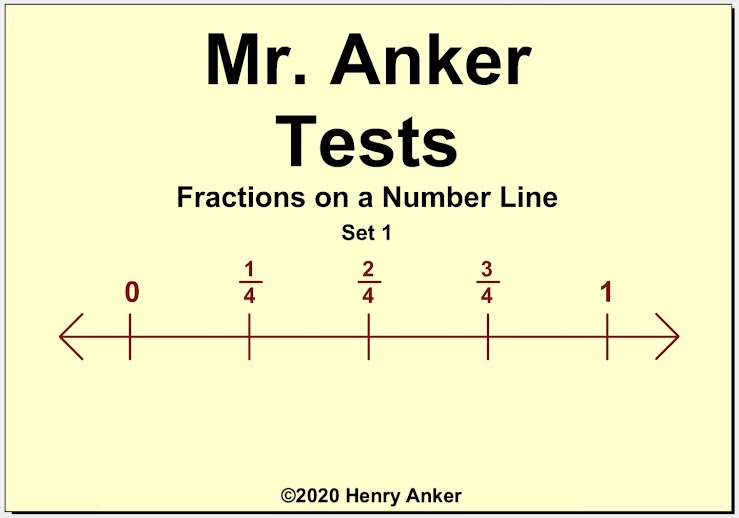Fractions on a Number Line Help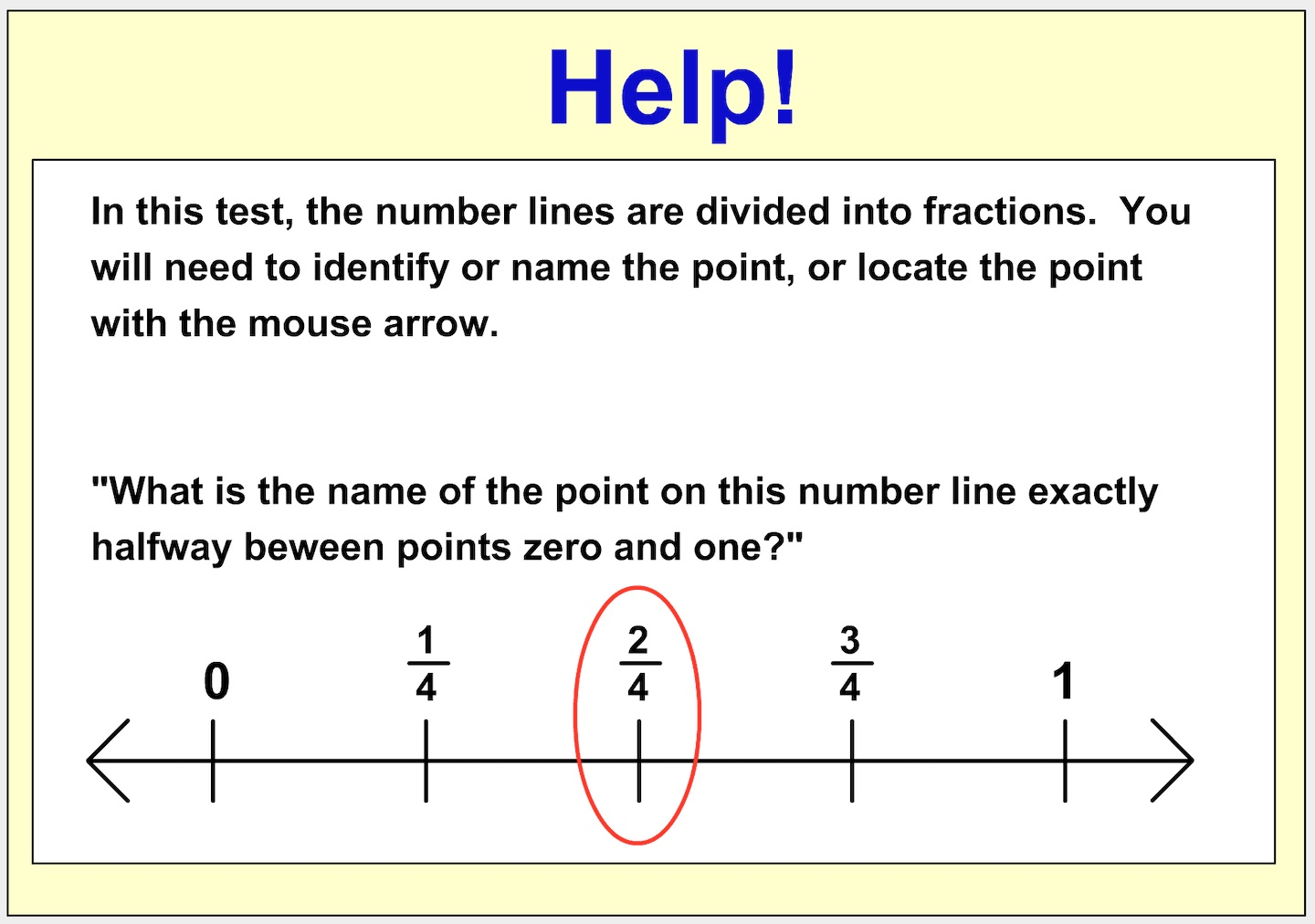1.  Point K is in which position on the number line? *
1 point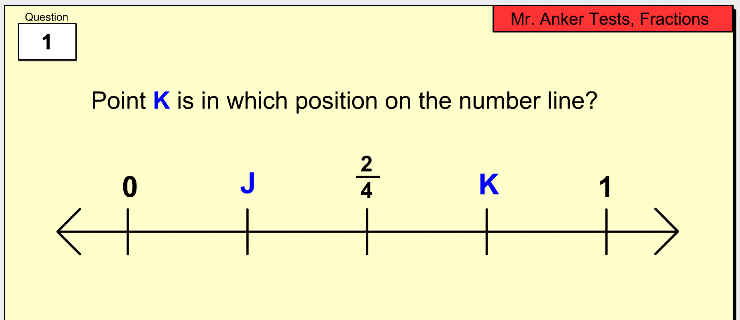2.  Point T is in which position on the number line? *
1 point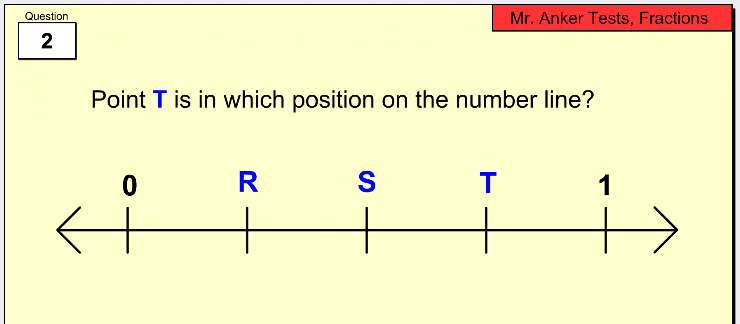3. Point C is in which position on the number line? *
1 point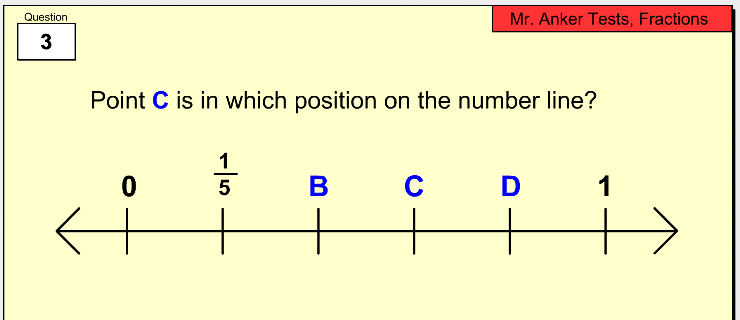4.  Which lettered point is found at 2/4 on the number line? *
1 point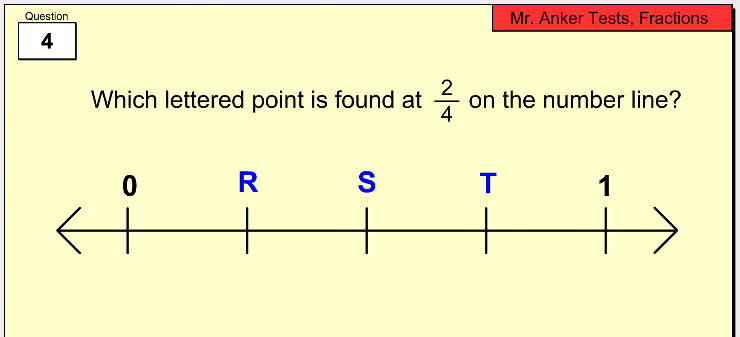5.  What is the correct name for Point S on the number line? *
1 point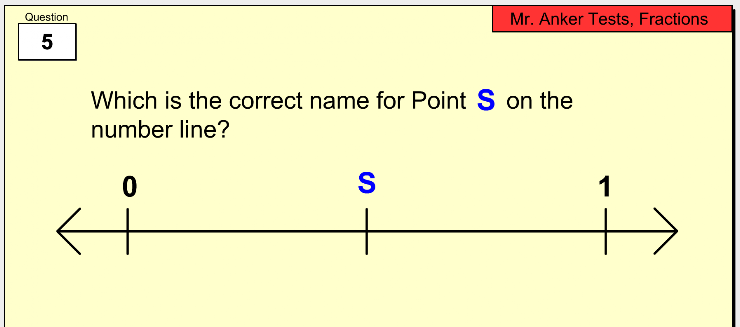6. How is this number line divided? *
1 point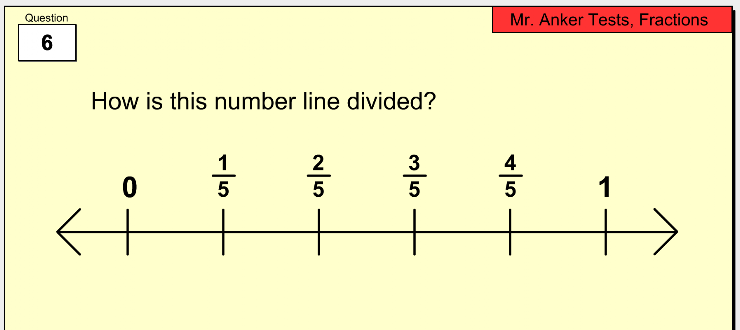7. Which of the statements below is false? *
1 point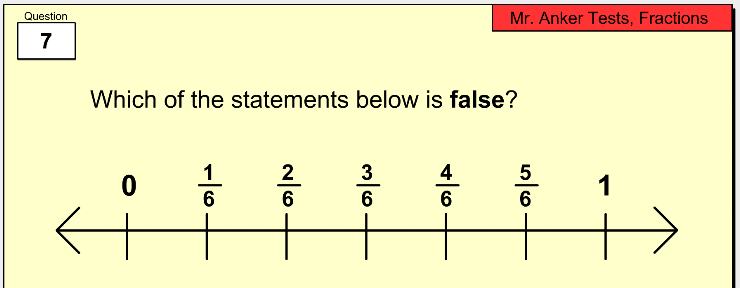8. Which point represents 1 & 1/2 on the number line? *
1 point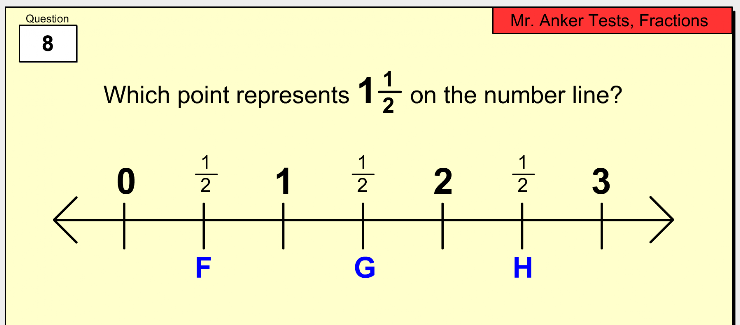9. What fraction does Point W represent on the number line? *
1 point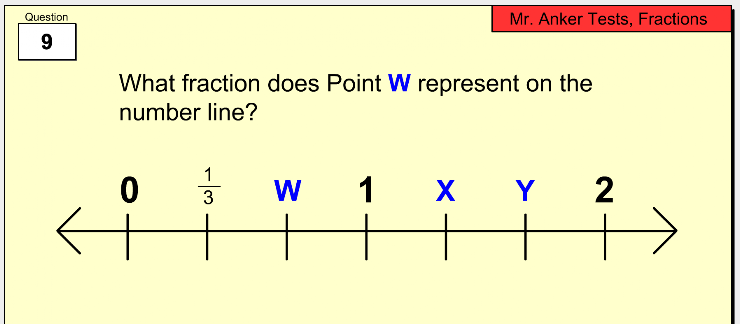10. Point Y is in which position on the number line? *
1 point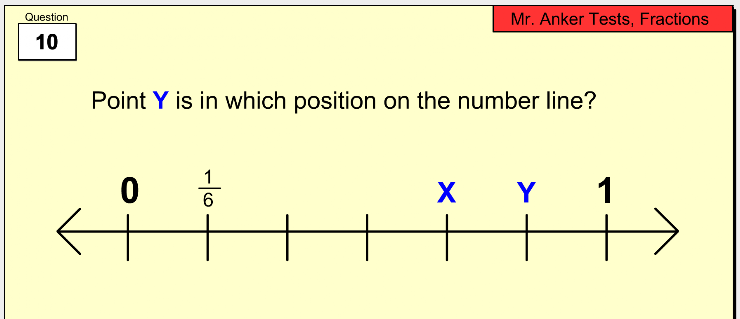11. What mixed number does Point Y represent on the number line? *
1 point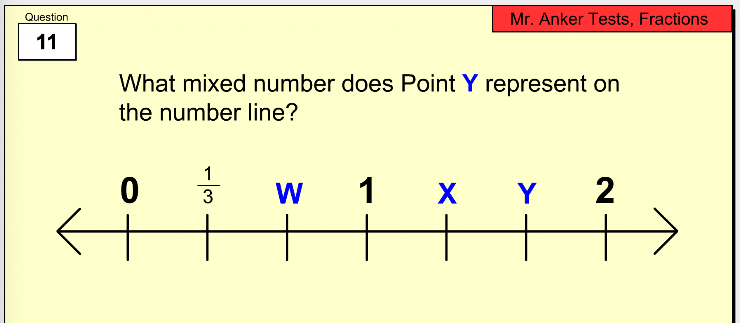12. What mixed number or whole number does Point D represent on the number line? *
1 point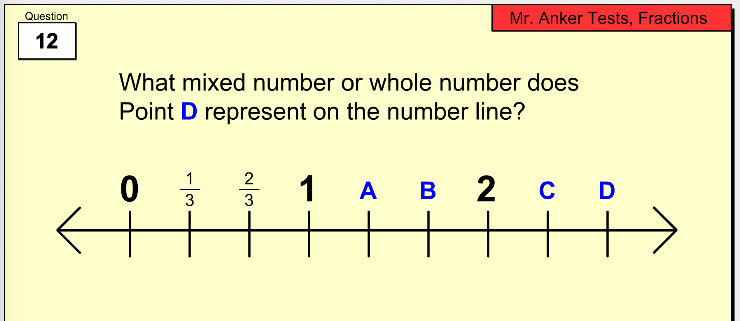13. Which letter represents a whole number on the number line? *
1 point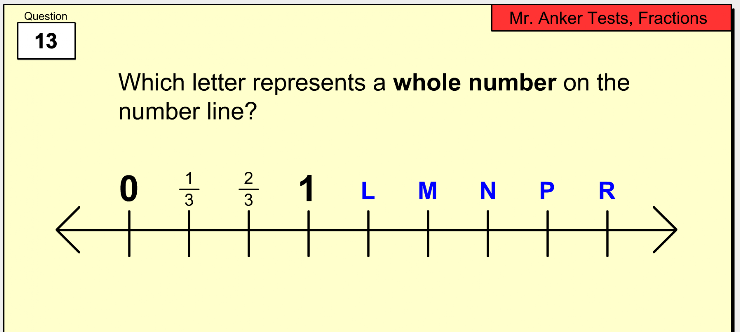14. Which fraction or mixed number does Point F represent on the number line? *
1 point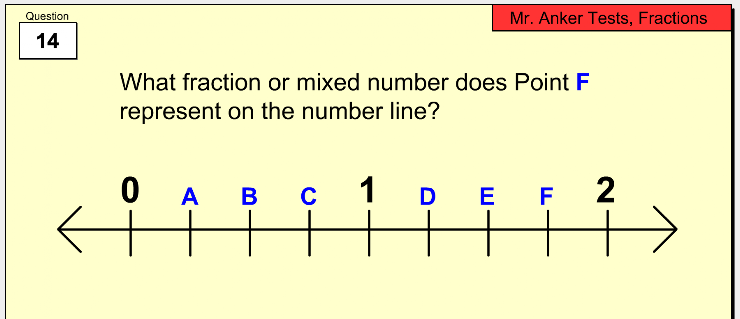15. Which lettered point represents a mixed number on the number line? *
1 point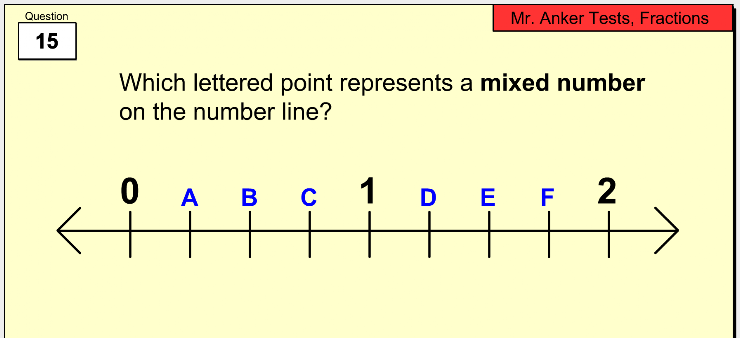16.  Which lettered point is located exactly halfway between 0 and 1 on the number line? *
1 point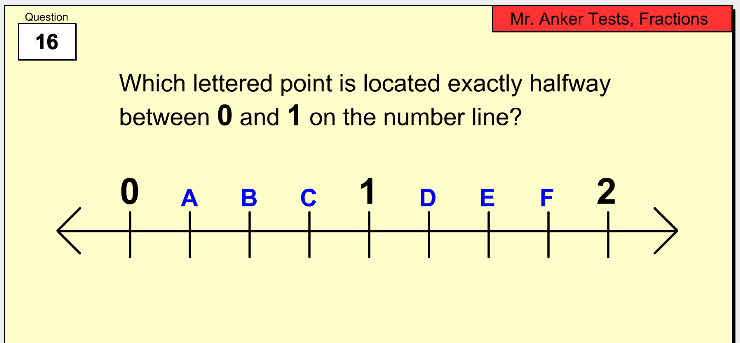17. Which lettered point represents the mixed number 4 & 1/2 on the number line? *
1 point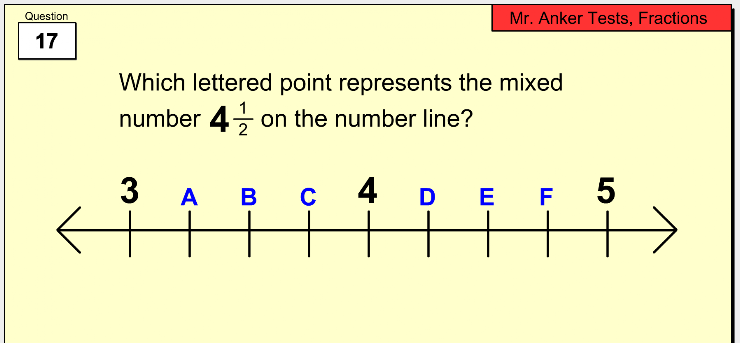18.  From the dropdown menu, choose the correct way to complete this statement:  "Three-eighths is _____________ one-fourth." *
1 point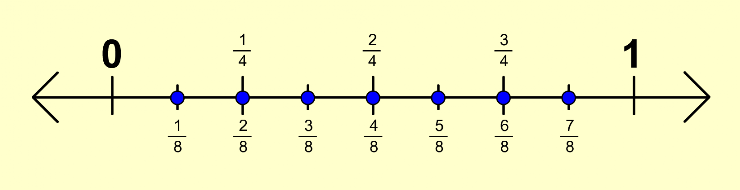19. From the dropdown menu, choose the correct way to complete this statement: "Four-sixths is _____________ two-thirds." *
1 point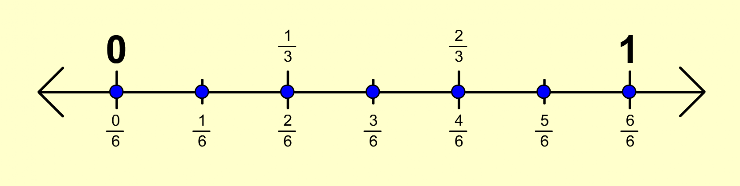20. From the dropdown menu, choose the correct way to complete this statement: "The whole number one is _____________ the fraction six-sixths." *
1 point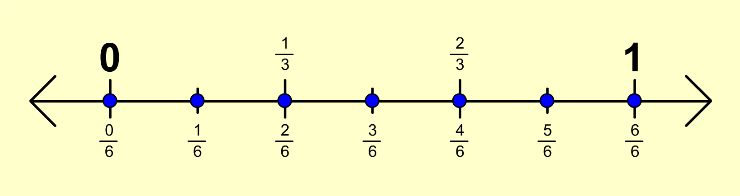Submit
Clear form